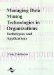# Individual Forecasting Methods

 < Day Day Up >

A total of 11 different statistical forecasting methods were considered in this research for forecasting the univariate series. They were chosen because they are popular time series forecasting methods and they are also relatively easy to use and can easily be found in common forecasting packages. They were grouped into three univariate forecasting procedures: exponential smoothing, box-jenkins, and regression over time. There were three alternatives under exponential smoothing and four alternatives under Box-Jenkins and regression over time, as given in Table 2. Only one method from each procedure was included in the linear combination. This was done on the supposition that the three groups of methods were different classes of forecasting model and thus might contribute something distinct, whilst there was likely to be much less extra contribution from different methods within the class.

Table 2: The alternative forecasting methods in each procedure

Exponential Smoothing

Box-Jenkins

Regression Over Time

Simple exponential

AR(1)

linear

AR(3)

power

Holt's method, exponential smoothing with trend

MA(1)

logarithmic

MA(3)

hyperbolic

The 11 forecasting methods included by this research are simple time series models. These methods are selected for the combination in an equitable fashion to all the 10 series considered. This is because all the 10 series are of similar nature (monthly demands of forms) and all the forecasts in this research are one-step-ahead. It might be useful to attempt to tailor the choice of methods to particular situations. For example, Lewandowski's FORSYS system, in the M-competition (Makridakis et al., 1982) appears to be particularly valuable for long forecast horizons. Thus it might be a prime candidate for inclusion in situations with long horizons but not necessarily in situations with short horizons. It is also important to note that combining forecasts is not confined to combination utilizing time series methods as was the case in this research. The desire to consider any and all available information means that forecasts from different types of sources should be considered. For example, one could combine forecasts from time series methods with forecasts from econometric models and with subjective forecasts from experts.

The first 36 data points were used to build the model and determine the best set of parameters for each of the forecasting methods and then to select which of the methods gave the best forecasting results for that procedure group, based on the usual mean squared error criterion.

 < Day Day Up >Managing Data Mining Technologies in Organizations: Techniques and Applications
ISBN: 1591400570
EAN: 2147483647
Year: 2003
Pages: 174